Question

The total cost (in dollars) for a company to manufacture and sell x items per week is C=40x+180, whereas the revenue brought in by selling all x items is R=68x−0.4×2. How many items must be sold to obtain a weekly profit of $300? in progress 0 5 months 2021-08-31T05:46:58+00:00 1 Answers 7 views 0 ## Answers ( ) 1. Answer: The company needs to sell either 30 or 40 items. Step-by-step explanation: We are given that the cost for selling x items given by the function: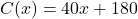And the revenue for selling x items is given by: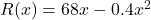The profit function is the cost function subtracted from the revenue function: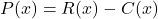Substitute and simplify: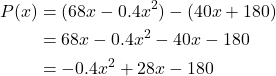To find how many items must be sold in order to obtain a weekly profit of$300, we can let P equal 300 and solve for x. So: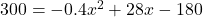Solve for x. Subtract 300 from both sides: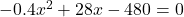We can divide both sides by -0.4: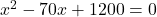Factor: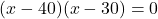Zero Product Property: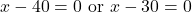Solve for each case: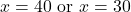So, in order to obtain a weekly profit of \$300, the company need to sell either 30 or 40 items.